Latest Banking jobs   »

# Quantitative Aptitude Quiz For IBPS RRB PO/Clerk Prelims 2023 -02nd June

Q1. If ratio between volume of a cylinder and volume of sphere is 3 : 1, then find the ratio between total surface area of cylinder and total surface area of sphere [Note: Radius of sphere = Radius of cylinder]
(a) 2 : 1
(b) 5 : 2
(c) 4 : 1
(d) 3 : 2
(e) 7 : 2

Q2.The side of a square is 25% more than the side of an equilateral triangle whose perimeter is 48 m. Find the ratio of area of square to area of triangle.
(a) 25 : 12
(b) 25√2 : 12
(c) 25√3 : 12
(d) 20√3 : 12
(e) 24 √3 : 12

Q3. A rectangular field cost Rs. 110 for leveling at 50 paise per square meter. If the ratio of length to breadth is 11: 5. Find the breadth of field?
(a) 12 m
(b) 10 m
(c) 5 m
(d) 16 m
(e) 15 m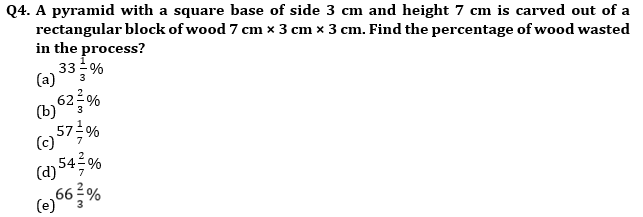Q5. The length of rectangular field is thrice its breadth. If Rs 480 is required to paint the floor at the rate Rs 2.5 per sq m, then what would be the difference between the length and breadth of the field?
(a) 16 m
(b) 8 m
(c) 12 m
(d) 24 m
(e) 20 m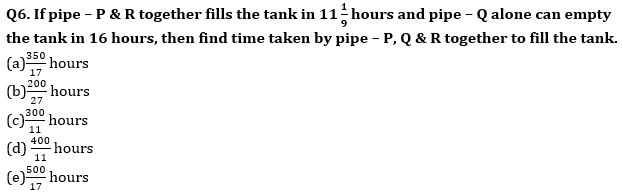Q7. CP of article – A is 80% of that of article –B. Article – A is marked 60% above its CP and 20% discount is allowed on it. If discount allowed on article – A is Rs.20 more than profit earned on article – A, then find CP of article – B.
(a) Rs.600
(b) Rs.650
(c) Rs.675
(d) Rs.575
(e) Rs.625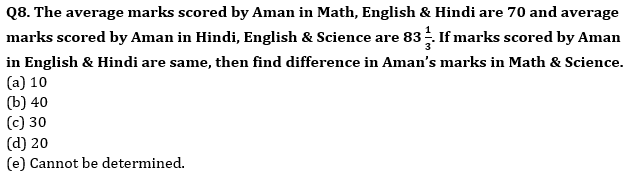Q9. A team of 5 persons consisting of 3 male and 2 female is to be formed out of 8 male and 5 female. Find in how many ways the team can be formed?
(a) 560 ways
(b) 840 ways
(c) 1280 ways
(d) 40 ways
(e) 1287 ways

Q10. Train – B can cross train – A in 90 seconds while running in same direction. Ratio of length of train – A to that of B is 4 : 5 and difference in speed of train – A & B is 36 km/hr. If train – A can cross 200m long platform in 24 seconds, then find speed of train – B.
(a) 162 km/hr.
(b) 90 km/hr.
(c) 144 km/hr.
(d) None of the above options.
(e) Cannot be determined.

Solutions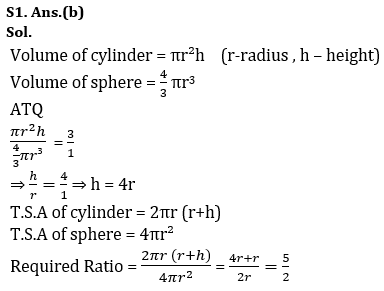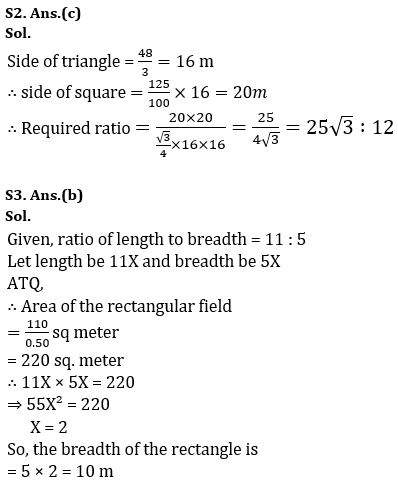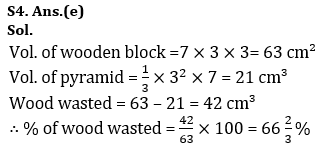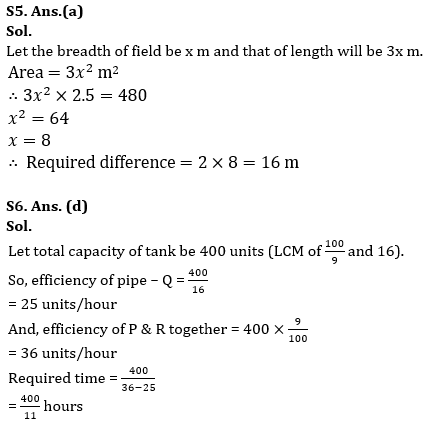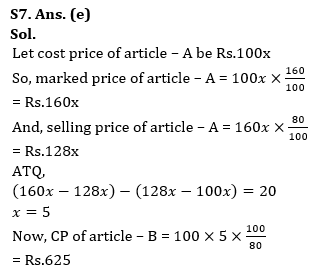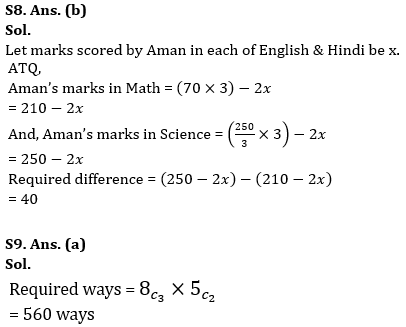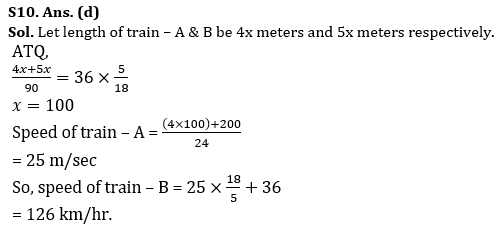.                                                                                                         .

## FAQs

### What is the selection process of IBPS RRP PO?

The Selection Process of IBPS RRB PO consists of Prelims, Mains and Interview

#### Congratulations!Union Budget 2023-24: Free PDF